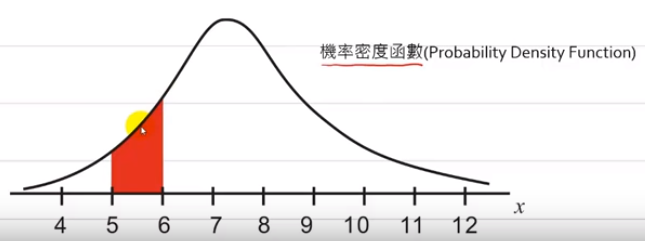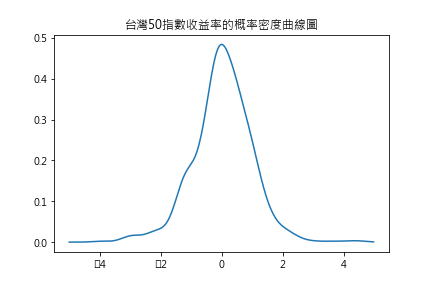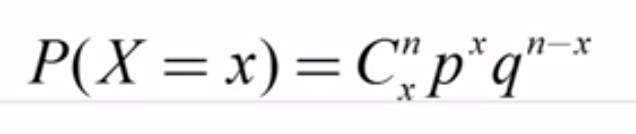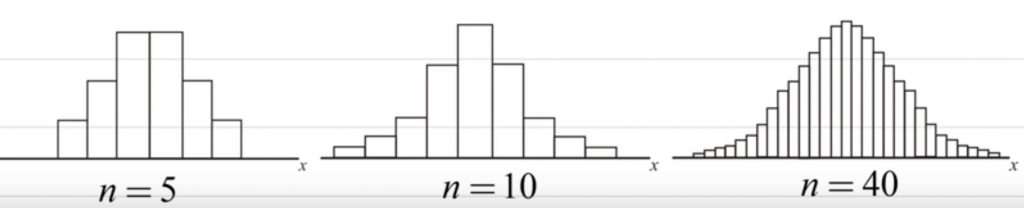#2019 iT 邦幫忙鐵人賽DAY 21
1
AI & Data

## [Day21]統計學基礎 - 隨機變數(Random Variable)

### 前言

1. 離散型隨機變數(Discrete Random Variable)：隨機變數取值的範圍有限個(ex 擲硬幣只有兩種結果，所以取擲的範圍有限制)
2. 連續型隨機變數(Continuous Random Variable)：隨機變數可以在一個區間上任意取值(ex 股票中的收益率就是連續型隨機變數)

### 離散型隨機變數(Discrete Random Variable)：

``````choice(a, size=None, p=None)
``````

a：隨機變數所有可能的取值
size：產生的陣列大小
p：與a同等大小，指定每種數據發生的機率

``````import numpy as np
import pandas as pd

ironman = np.random.choice([1,2,3,4,5], size=1000, p=[0.25,0.1,0.35,0.2,0.1])
valueCount = pd.Series(ironman).value_counts()
print('valueCount/1000---->', valueCount/1000)
``````
``````# 輸出結果
valueCount/1000----> 3    0.365
1    0.245
4    0.191
5    0.119
2    0.080
``````

### 連續型隨機變數(Continuous Random Variable)：``````from scipy import stats
import matplotlib.pyplot as plt
%matplotlib inline

tw50_gaussian = stats.kde.gaussian_kde(tw50.ROI)
# gaussian_kde-> 高斯核函數，kde 是核函數密度估計
x_value = np.arange(-5,5,0.02)

fig = plt.figure()
plt.subplot()
plt.plot(x_value, tw50_gaussian(x_value))
plt.title("台灣50指數收益率的概率密度曲線圖")
``````### 二項分佈Numpy中產生二項分佈隨機數的函式如下：

``````binomial(n,p,size)
``````

n：二項實驗的次數
p: 表示成功的機率
size: 隨機產生的數量

``````np.random.binomial(100,0.5,20)
``````
``````# 輸出結果
array([56, 49, 49, 52, 44, 50, 40, 52, 46, 49, 54, 46, 55, 49, 57, 45, 45,
54, 53, 47])
``````

``````from scipy import stats
stats.binom.pmf(20,100,0.5)
``````
``````# 輸出結果
4.228163267601253e-10
``````

``````ret = tw50.ROI  #台灣50的收益率數據
ret.index = pd.to_datetime(tw50.index)
ret1 = ret['2016']

#台灣50上漲的機率p
p = len(ret1[ret>0]) /len(ret1)
print('p----->', p)

#預估未來20個交易日中，有7個是上漲的機率
prob =stats.binom.pmf(6,20,p)
print('prob----->', prob)
``````
``````# 輸出結果
p-----> 0.5327868852459017
prob-----> 0.02093703958512302
``````

### 常態分佈### 之前的章節

• 安裝環境
• Seaborn
• 統計
• 程式碼位置
• github
因為作者本身也是第一次學習Python和寫程式文章，所以編排上會有點亂，觀念可能也會錯誤，如果有疑問可以提出一起討論。

python 入門到分析股市30

### 1 則留言

0

Summer iT邦新手 5 級‧ 2019-05-07 11:48:44 檢舉